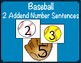Subject
Resource Type
File Type

PDF

(16 MB|51 pages)
Standards
Also included in:
1. Do you need some baseball themed literacy and math activities? These resources are for you! Easy Prep Baseball Bundle With Cross-Curricular Centers & Activities for Lower Elementary Children! This bundle includes both literacy and math resources for kindergarten and first grade students. Basebal
\$50.00
\$40.00
Save \$10.00
• Product Description
• StandardsNEW

Do you need a baseball themed math activity? This resource is for you! Baseball Build a 2 Addend Addition or Subtraction Number Sentences. Have your students practice their 2 addend addition and subtraction math facts with this center or small group game. Baseball fun for everyone!

Resource Prep: Print, laminate and cut all cards and pieces to the activity. You can cut out the individual cards or leave them as square cards. Directions are also located on the game card set. Note: You can choose to do all the cards or take out the number of cards you are working on at the time.

Resource Includes the Following:

☆ 122 Card Sets: 0-20 Addition & Subtraction Facts

☆ Baseball Build an Addition Number Sentence Mat: 2 Types

☆ Baseball Build a Subtraction Number Sentence Mat: 2 Types

☆ Baseball Build an Addition Sentence Number Worksheet

☆ Baseball Build a Subtraction Number Sentence Worksheet

Resource Directions:

Baseball Build a 2 Addend Addition Number Sentence: Addition: Children can begin with either of the baseballs to begin their number sentence. Children must have each of the cards to build their number sentences. Ex: 1st Addend white baseball : 1; 2nd addend yellow baseball: 2; and then the card: 3; 1 + 2=3. Children can stack the cards together.

Baseball Build a 2 Addend Subtraction Number Sentence: Subtraction: Children begin with Glove Card and build their number sentence. Children must have each of the cards to make the number sentence. Ex: Minuend Glove Card : 3, either of the baseball cards as their subtrahend, yellow baseball: 2 and then the difference the card that they had not used, white baseball: 1; 3 - 2=1. Children can stack the cards together.

Baseball Build an Addition Number Sentence Mat & Build a Subtraction Number Sentence Mat: Children can use these mats to help organize how to make a number sentence. A good resource for students who are just learning number sentences or for those students who need visual aids.

Baseball Build an Addition Sentence Number Worksheet & Build a Subtraction Number Sentence Worksheet: Children can use these worksheets to record their work and as an assessment piece for teachers to see if students are understanding the concepts.

Kids have some baseball fun while learning two addend addition and math facts!

✤ Please check out the preview for this product.

✤ Don’t forget to leave feedback. You will receive TPT credits that can be used on your future purchases!

Related Products

Baseball Math Centers

Baseball Literacy Centers

Spring Themed Literacy & Math Bundle

Spring Unit: Activities & Center

Add and subtract within 20, demonstrating fluency for addition and subtraction within 10. Use strategies such as counting on; making ten (e.g., 8 + 6 = 8 + 2 + 4 = 10 + 4 = 14); decomposing a number leading to a ten (e.g., 13 - 4 = 13 - 3 - 1 = 10 - 1 = 9); using the relationship between addition and subtraction (e.g., knowing that 8 + 4 = 12, one knows 12 - 8 = 4); and creating equivalent but easier or known sums (e.g., adding 6 + 7 by creating the known equivalent 6 + 6 + 1 = 12 + 1 = 13).
Relate counting to addition and subtraction (e.g., by counting on 2 to add 2).
Understand subtraction as an unknown-addend problem. For example, subtract 10 – 8 by finding the number that makes 10 when added to 8.
Apply properties of operations as strategies to add and subtract. If 8 + 3 = 11 is known, then 3 + 8 = 11 is also known. (Commutative property of addition.) To add 2 + 6 + 4, the second two numbers can be added to make a ten, so 2 + 6 + 4 = 2 + 10 = 12. (Associative property of addition.)
Fluently add and subtract within 5.
Total Pages
51 pages
N/A
Teaching Duration
N/A
Report this Resource to TpT
Reported resources will be reviewed by our team. Report this resource to let us know if this resource violates TpT’s content guidelines.